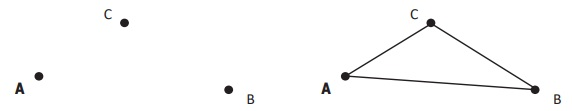Home | | Maths 6th Std | Basic Elements of a Triangle

# Basic Elements of a Triangle

Mark 3 points A, B, C on a paper, such that they do not lie on a straight line. Join the line segments AB, BC and CA.

Basic Elements of a Triangle

Mark 3 points A, B, C on a paper, such that they do not lie on a straight line. Join the line segments AB, BC and CA.This forms a triangle ABC represented as Δ ABC or Δ BCA or Δ CAB.

In Δ ABC, the line segments AB, BC and CA are called the sides of the triangle and CAB, ABC and BCA (A, B & C) are called the angles of the triangle. The point of intersection of two sides of the triangle is called the vertex. A, B and C are three vertices of ∆ ABC. Hence, a triangle has 3 sides, 3 angles and 3 vertices.

Think

Can a triangle be drawn using 3 points  on a straight lineTags : Geometry | Term 2 Chapter 4 | 6th Maths , 6th Maths : Term 2 Unit 4 : Geometry
Study Material, Lecturing Notes, Assignment, Reference, Wiki description explanation, brief detail
6th Maths : Term 2 Unit 4 : Geometry : Basic Elements of a Triangle | Geometry | Term 2 Chapter 4 | 6th Maths